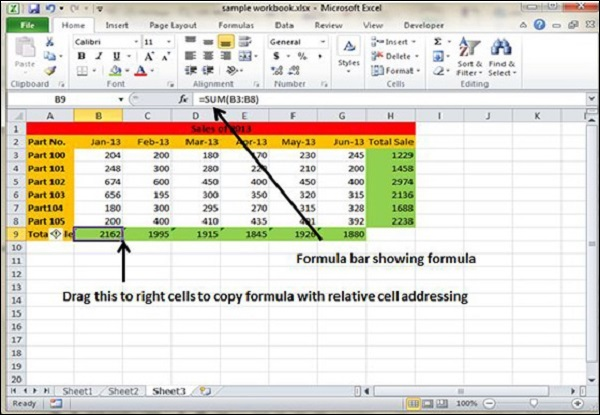# Copying Formulas in Excel 2010

## Copying Formulas in MS Excel

Copying formulas is one of the most common tasks that you do in a typical spreadsheet that relies primarily on formulas. When a formula uses cell references rather than constant values, Excel makes the task of copying an original formula to every place that requires a similar formula.

MS Excel does it automatically adjusting the cell references in the original formula to suit the position of the copies that you make. It does this through a system known as relative cell addresses, where by the column references in the cell address in the formula change to suit their new column position and the row references change to suit their new row position.

Let us see this with the help of example. Suppose we want the sum of all the rows at last, then we will write a formula for first column i.e. B. We want sum of the rows from 3 to 8 in the 9th row.After writing formula in the 9th row, we can drag it to remaining columns and the formula gets copied. After dragging we can see the formula in the remaining columns as below.

• column C : =SUM(C3:C8)

• column D : =SUM(D3:D8)

• column E : =SUM(E3:E8)

• column F : =SUM(F3:F8)

• column G : =SUM(G3:G8)